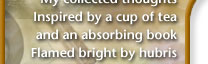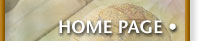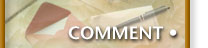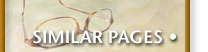## Clustered Random Numbers

One aspect of functional programming is the use of standard functions. For this little example, I used CoffeeScript, however, you should be able to translate it easily in JavaScript, or just look at the compiled source code. Of course, I don't mean we have some sort of standards body or anything official, it is just that over the years, we can expect certain useful functions to be available to the functional programmer.

Recently, I wanted to program some art in order to show my kids the HTML5 canvas, and came up with this:

Your browser doesn't support HTML5's Canvas…

As you can tell, the color choices for each circle is random, but not evenly distributed…a collection of color choices clustered around a single value. For instance, the colors are mostly similar (like reds), but with occasional greens or yellows††Refresh this page to have another goal color chosen .

In other words, we want a bell curve random number generator. The way I did it uses the accepted functions from the Underscore library.

## Data Generator

To begin our experiments, let's first make a data collector function that takes a sample size and some random number generator that we want to test, and returns the actual results of running the generator:

``````data_generator = (size, generator) ->
_.map( _.groupBy( _.times(size, generator)), _.size )
``````

Since doing all work by using a standard functional library may be unfamiliar, let's pick this line apart starting from the inside…

• times simply generates an array of `size` elements, where each value is a call to the `generator` function. If we pass it a six-sided die function, the values would all be from `1` to `6`.

• groupBy counts the number of values of particular type. For instance is `size` is `10`, we might end up with:

``````{ '1': [ 1 ],
'3': [ 3, 3, 3, 3 ],
'4': [ 4, 4 ],
'5': [ 5, 5 ],
'6': [ 6 ]
}
``````
• map loops through the 6 entries in the object calls the size function. The first entry in the resulting array is the values of all the 1's, the second entry is all the 2's, etc.

The beauty of functions like these is that you can use them in any language. Granted, the `map` function isn't very clear, and in CoffeeScript, we often can use a loop comprehension, however, comprehensions don't handle with objects as well as the `map` function.

You could write our one-line call to the Underscore library in an iterative way, but once you get the hang of these functions, transforming data becomes more clear than something like:

``````function data_generator(size, generator) {
var data = {};
for (var i = 0; i < size; i++) {
var roll = generator();
if ( data[roll] ) {
data[roll]++;
}
else {
data[roll] = 1;
}
}
return data;
}
``````

## Rolling One Die

A dice rolling function can be done with a call to `Math.floor(Math.random() * 6) + 1`, but Underscore has a random function that is more clear:

``````die = -> _.random(1, 6)
``````

Either way, we now can generate some data by calling:

``````data_generator(10000, die)
``````

Which returns (at least, one time):

1. `1697`
2. `1692`
3. `1657`
4. `1629`
5. `1700`
6. `1625`

Where each value should be around `1667`, or 1/6th of the total. This test shows that we are getting a fairly even distribution between the six choices.

## Rolling Two Dice

If you roll two 6-sided dice, we can easily predict the values for any particular roll based on the number of combinations.

Let's try an actual experiment:

``````dice2 = -> _.random(1,6) + _.random(1,6)
``````

We can compare the probability with an actual run:

Roll Results Probability
2 259 1/36
3 571 2/36 or 1/18
4 805 3/36 or 1/12
5 1086 4/36 or 1/9
6 1392 5/36
7 1660 6/36 or 1/6
8 1413 5/36
9 1142 4/36 or 1/9
10 817 3/36 or 1/12
11 568 2/36 or 1/18
12 287 1/36

Graphing this demonstrates the values cluster in the middle, around 7, but the sides are pretty straight: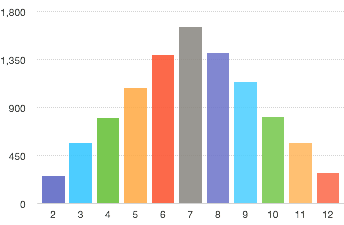## Rolling More Dice

However, I want more of a bell curve. Easy… just add more dice. How many? Well, we can make a general function for rolling as many dice as we want:

``````roll = (how_many) -> sum( _.times(how_many, die) )
``````

The `roll` function calls the times function to roll our a few dice by calling our `die` function and adding all the results (of the array) by calling this `sum` function:

``````add = (x,y) -> x + y

sum = (lst) -> _.reduce(lst, add, 0)
``````

The reduce function is a bit more complicated to understand, but pretty powerful. Essentially, it reads each element in the `lst` array and calls a function, `add` on it with an accumulator, which starts at `0`. So, it adds `0` with the first element in the array, and then it adds the second number to that result, etc.

So, we can define a 3 or 4-die roll:

``````dice3 = -> roll(3)
dice4 = -> roll(4)
``````

Running `_generator(10000, dice4)` and graphing the resulting data shows a definite tendency to the middle: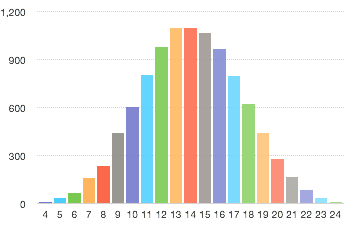## Rolling Within a Range

Now, we have the idea, let's make it more versatile and allow us to pick a better range of numbers. For instance, if we wanted numbers up to 100, and we are rolling 4 dice, we just have to make sure those dice have 25 sides each.

While not physically possible, that is easy to render in our computer. Wait, if we want a range from 1 to 100, we don't want four 25-sided dice, we want four 26-sided dice, and then remember to subtract 4…

``````  roller = (range) ->
sides = (range + 4) / 4
dice = -> _.random( 1, sides )
sum( _.times(steep, dice) ) - 4;
``````

But four dice is actually pretty flat, and we'll want to experiment with the steepness of our curve, so we should refactor the `4` into a `steep` variable.

## Shift Top of Bell from Middle

Having the top of the bell near some other number, is a simple shift of the values. We just need to figure out the amount to shift by first figuring out the distance from the middle to the goal:

``````  goal_roll = (goal, range) ->
steep = 20        # Yeah, I found this to be the best

sides = (range + steep) / steep
delta = goal - range / 2

dice = -> _.random( 1, sides )

roll = sum( _.times(steep, dice) ) -steep + delta
if roll > range
roll - range  # This approach works well with
else              # colors that wrap around a range
roll
``````

Done! I can now use that as the basis for a color choosing algorithm for the above artwork. Hope this gives you some ideas how functional programmers expect to build on top of a library of expected functions, like the Underscore library.